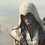# About conservation of charge

We studied that charge is conserved and mass is not conserved.suppose there is a electron with mass 9.10938291 × 10-31 kilograms and charge 1.60217657 × 10-19 coulombs.if we convert the whole the mass into energy what will happen to the charge?we have also studied that mass-less object can't carry charge.Note by Sreehari Vp
5 years, 10 months ago

MarkdownAppears as
*italics* or _italics_ italics
**bold** or __bold__ bold
- bulleted- list
• bulleted
• list
1. numbered2. list
1. numbered
2. list
Note: you must add a full line of space before and after lists for them to show up correctly
paragraph 1paragraph 2

paragraph 1

paragraph 2

[example link](https://brilliant.org)example link
> This is a quote
This is a quote
    # I indented these lines
# 4 spaces, and now they show
# up as a code block.

print "hello world"
# I indented these lines
# 4 spaces, and now they show
# up as a code block.

print "hello world"
MathAppears as
Remember to wrap math in $$...$$ or $...$ to ensure proper formatting.
2 \times 3 $$2 \times 3$$
2^{34} $$2^{34}$$
a_{i-1} $$a_{i-1}$$
\frac{2}{3} $$\frac{2}{3}$$
\sqrt{2} $$\sqrt{2}$$
\sum_{i=1}^3 $$\sum_{i=1}^3$$
\sin \theta $$\sin \theta$$
\boxed{123} $$\boxed{123}$$

Sort by:

what is the relation of mass with charge?

- 5 years, 10 months ago

I think there should be relation between mass and charge carried by the object obeying the energy conservation!!!!

- 5 years, 10 months ago

the charge will disappear with all the charges inside but with only a friction in the end some atomic nuclear (hypotenuse), E=mc^2 from the equation if you lose 1 gram of mass, it will create an energy greater that hiroshima nuclear bomb, the mass mostly come from neutron wich is neutral and only by losing the mass of the neutron can be converted to ''high energy density'', how about electron? the mass to given an energy is to ''small''.

- 5 years, 10 months ago

i guess if we use all the energies(mass energy equivalence) inside the body the body dismantles and its no more the mass...so is the charge!

- 5 years, 10 months ago

electron is a fundamental particle with a negative charge. to convert all of electron's mass to energy, one has to react the electron with the antimatter equivalent of the electron, which is a positron. A positron carries a positive charge. as such, charge is still conserved.

- 5 years, 10 months ago

is there any other method to convert electron into energy? can't we make energy,E=MeC^2(Me=mass of electron)from an electron?throw this method we get energy,E=2MeC^2

- 5 years, 10 months ago

The short answer: you can't. That's the beauty of physics. In order to uphold both the conservation of charge and the energy-mass equivalence, nature is such that the electron must annihilate with another particle with the opposite charge.

- 5 years, 10 months ago

then how energy is producing in nuclear reactors?

- 5 years, 10 months ago

the mass of the products is less than the mass of the reactants. the nuclear equation is still balanced in terms of charge, mass number and atomic number. the mass defect is converted into energy.

- 5 years, 10 months ago

Nice answer Jiahai F.

- 5 years, 10 months ago

You have said that mass less objects cannot carry charge, can you please explain why is it so?

- 5 years, 10 months ago

if there is charge, there must be electrons or protons, and they carry charge.

- 5 years, 10 months ago

I don't know.........but i heard it from different places

- 5 years, 10 months ago

charge remains intact.using the mass energy equation we do only get the energy equivalent of mass which is the all form of energy stored in the body.charge is its intrinsic property

- 5 years, 10 months ago

Suppose somehow we did convert electron's mass into energy then what? Its theoretically possible I suppose. Is there a reason why it can't be otherwise.

- 5 years, 10 months ago

theoritically it is possible to calculate but its too less and can be ignored i guess.and the use of equivalence of mass energy is the total energy stored in the body and to use it to the fullest i guess research might be going on....and total energy just mean the potential,kinetic,nuclear,gravitational and all forms of energy engulfed within the body forming the shape of the matter....

- 5 years, 10 months ago

i didn't get the idea.please explain......

- 5 years, 10 months ago

charge is carried by electrons and protons. If you convert them to energy I guess you lose charge.

- 5 years, 10 months ago

but charge is conserved.

- 5 years, 10 months ago

does the body get disappear??if no then the charge will always be conserved...we just lose the mass

- 5 years, 10 months ago